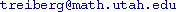Course Title: Riemannian Geometry Fall 2005 MATH 6170 - 1 Andrejs Treibergs MW 12:55-1:25 in LCB 225 MWF 10:45 - 11:35 in JWB 224.Some knowledge of differentiable manifolds (e.g. MATH 6510-6520 or consent of instructor.) Takashi Sakai, Riemannian Geometry, AMS 1996

This course is useful for students of geometry, topology, geometric group theory, nonlinear analysis, general relativity ands graphic design.

Abstract. Recent progress by Perelman on Hamilton's Program to solve Thurston's Geometriztion Conjecture, which would imply the Poincare Conjecture, has the mathematical world extremely excited. Topologists are optimistic that Perelman's arguments will completely hold up under expert scrutiny.

The objective of this course is to start from basic notions from Riemannian Geometry and build to Perelman's Theorems. How does curvature influence on the local and global geometry and topology of a manifold? We shall discuss basic examples such as hyperbolic space and Riemannian surfaces in considerable detail. The principal tool is to use the curvature behavior of geodesics, which are length minimizing curves. We shall develop the intrinsic, classical and differential form notations in parallel. Later we shall prepare some geometric analysis tools such as the Ricci Flow, a systematic deformation that splits a manifold into understandable pieces, used by Perelman. We shall loosely follow Sakai's text for the bulk of the course.

Topics. We shall loosely follow Sakai's text for the bulk of the course. Topics include (depending on time):

• Riemannian Metrics
• Connections. Affine & Riemannian.
• Geodesics. Minimizing properties of geodesics; convex neighborhoods; geodesic flow.
• Curvature. Sectional curvature; Ricci & scalar curvature; tensors.
• Jacobi fields. Conjugate points.
• Isometric Immersions. Second fundamental form.
• Complete manifolds. Hopf-Rinow Theorem; Hadamard Theorem.
• Space Forms. Cartan's Theorem on recovering the metric from curvature; hyperbolic space and its properties.
• Variation of Geodesics. First and second variation formulae for length and energy of geodesics. Theorems of Bonnet-Meyers-Synge.
• Index Theory of Geodesics. Sturm-Liouville Theory. Rauch Comparison Theorem. Hessian comparison theorem. Laplacian Comparison Theorem and applications.
• Closed Geodesics. Preissman's Theorem.
• Cut locus. Conjugate locus. Injectivity radius.
• Toponogov Theorem. Alexandrov's Theorem. Soul Theorem.
• Sphere Theorem.
• Gromov-Hausdorff convergence.
• Collapsing.
• Ricci Flow and Hamilton's Sphere Theorem.

Last updated: 8 / 31 / 5